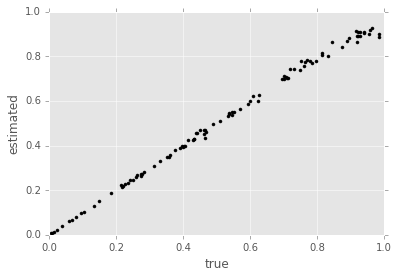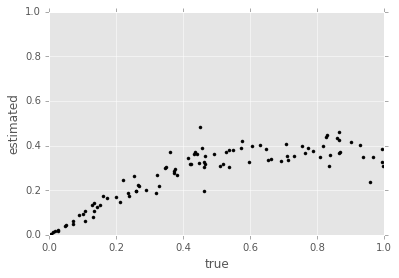# Multimodality and Resampling¶

This notebook tests the robustness of modified Liu-West (MLW) resampling to multimodality in the posterior distribution. We use as a test the familiar model $$\Pr(0 | \omega, t) = \cos^2(\omega t),$$ exploiting that the likelihood function is even in $\omega$ to produce posteriors that always have exactly two modes. Because this structure is not explicitly added to the resampling, but is easy to reason about analytically, it serves as a nice test case.

## Preamble¶

As usual, we start by configuring division and enabling plotting support.

In :
from __future__ import division, print_function
%matplotlib inline

In :
import numpy as np
import matplotlib.pyplot as plt
try: plt.style.use('ggplot')
except: pass


In order to view the incredibly verbose output from resampler debugging, it is helpful to point Python's logging functionality at a file instead of printing it inside the notebook. We use tempfile to pick a directory in a cross-platform way.

In :
import os, tempfile
logfile = os.path.join(tempfile.gettempdir(), 'multimodal_testing.log')
print("Logging to {}.".format(logfile))

import logging
logging.basicConfig(level=logging.DEBUG, filename=logfile)

Logging to C:\Users\Chris\AppData\Local\Temp\multimodal_testing.log.


Next, we import all the functionality from QInfer that we will need.

In :
import qinfer as qi

C:\Anaconda3\lib\site-packages\IPython\parallel.py:13: ShimWarning: The IPython.parallel package has been deprecated. You should import from ipyparallel instead.
"You should import from ipyparallel instead.", ShimWarning)
c:\users\chris\dropbox\software-projects\python-qinfer\src\qinfer\parallel.py:52: UserWarning: Could not import IPython parallel. Parallelization support will be disabled.
"Could not import IPython parallel. "


## Model and Prior Definition¶

We want the simple precession model $\cos^2(\omega t)$ to now extend over $\omega \in [-1, 1]$, so we redefine the are_models_valid method to allow for negative $\omega$.

In :
class NotQuiteSoSimplePrecessionModel(qi.SimplePrecessionModel):
@staticmethod
def are_models_valid(modelparams):
return (np.abs(modelparams) <= 1)[:, 0]


Having done so, we now set a prior that explicitly includes the degeneracy in the likelihood.

In :
model = NotQuiteSoSimplePrecessionModel()
prior = qi.UniformDistribution([-1, 1])

In :
def trial(a=0.98, h=None, track=True):
true_params = prior.sample()
updater = qi.SMCUpdater(model, 1000, prior, resampler=qi.LiuWestResampler(a=a, h=h, debug=True), track_resampling_divergence=track, debug_resampling=True)

for idx_exp in range(100):
exp = np.array([(9/8)**idx_exp], dtype=model.expparams_dtype)
datum = model.simulate_experiment(true_params, exp)

updater.update(datum, exp)

# Since this model is always degenerate about the origin, take the mean
# not of the original particles, but of the absolute value of the particles.
updater.particle_locations = np.abs(updater.particle_locations)

if track:
return [true_params, updater.est_mean()], np.mean(updater.resampling_divergences)
else:
return [true_params, updater.est_mean()]

In :
def corr(a=0.98, h=None, track=True, n_trials=100):
trues = np.zeros((n_trials,))
ests  = np.zeros((n_trials,))

if track:
divs = np.zeros((n_trials, ))

for idx_trial in range(n_trials):
if track:
print(idx_trial, end=' ')
(true, est), div = trial(a, h, track)
else:
true, est = trial(a, h, track)
trues[idx_trial] = true
ests[idx_trial] = est
if track:
divs[idx_trial] = div

if track:
return trues, ests, divs
else:
return trues, ests

In :
trues, ests = corr(1, 0.02, False, 100)

In :
bias = np.mean(np.abs(trues) - np.abs(ests))
risk = np.mean(np.abs(np.abs(trues) - np.abs(ests)))
print(bias, risk)

0.00697411114983 0.0123620858504

In :
plt.plot(np.abs(trues), ests, 'k.')
plt.xlabel('true')
plt.ylabel('estimated')
plt.xlim((0, 1))
plt.ylim((0, 1))

Out:
(0, 1)In :
trues, ests = corr(0.98, None, False, 100)
bias = np.mean(np.abs(trues) - np.abs(ests))
print(bias)

0.19281919156

In :
plt.plot(np.abs(trues), ests, 'k.')
plt.xlabel('true')
plt.ylabel('estimated')
plt.xlim((0, 1))
plt.ylim((0, 1))

Out:
(0, 1)## WIP: Mutliparameter Models¶

We also want to try with a multicos model, since that model admits a degeneracy between parameters that is broken by experimental variety. To arrive at a good estimate, then, a resampler must preserve unusual posterior structures that arise in the approach to a unimodal final posterior.

It's worth noting here that the traditional LW parameters work quite well for a better experimental protocol (choosing lots of varied experiments), but that this protocol may not always be available. Thus, MLW is a resource here to compensate for experimental restrictions.

In :
class MultiCosModel(qi.FiniteOutcomeModel):

def __init__(self, n_terms=2):
self._n_terms = n_terms
super(MultiCosModel, self).__init__()

@property
def n_modelparams(self):
return self._n_terms

@property
def is_n_outcomes_constant(self):
return True
def n_outcomes(self, expparams):
return 2

def are_models_valid(self, modelparams):
return np.all(np.logical_and(modelparams > 0, modelparams <= 1), axis=1)

@property
def expparams_dtype(self):
return [('ts', '{}float'.format(self._n_terms))]

def likelihood(self, outcomes, modelparams, expparams):
# We first call the superclass method, which basically
# just makes sure that call count diagnostics are properly
# logged.
super(MultiCosModel, self).likelihood(outcomes, modelparams, expparams)

# Next, since we have a two-outcome model, everything is defined by
# Pr(0 | modelparams; expparams), so we find the probability of 0
# for each model and each experiment.
#
# We do so by taking a product along the modelparam index (len 2,
# indicating omega_1 or omega_2), then squaring the result.
pr0 = np.prod(
np.cos(
# shape (n_models, 1, 2)
modelparams[:, np.newaxis, :] *
# shape (n_experiments, 2)
expparams['ts']
), # <- broadcasts to shape (n_models, n_experiments, 2).
axis=2 # <- product over the final index (len 2)
) ** 2 # square each element

# Now we use pr0_to_likelihood_array to turn this two index array
# above into the form expected by SMCUpdater and other consumers
# of likelihood().
return qi.FiniteOutcomeModel.pr0_to_likelihood_array(outcomes, pr0)

In :
mp_model = qi.BinomialModel(MultiCosModel(3))
mp_prior = qi.UniformDistribution([[0, 1]]*3)

In :
def multicos_trial(a, h, n_exps=200):
true = mp_prior.sample()
mp_updater = qi.SMCUpdater(mp_model, 2000, mp_prior,
resampler=qi.LiuWestResampler(a=a, h=h, debug=True),
debug_resampling=True
)

while True:
exp = np.array([(20 * np.pi * np.random.random(3), 40)], dtype=mp_model.expparams_dtype)
# To test the resampler, we take data according to one experiment design until it has to resample, then switch.
# Since the MLW resampler works quite well, we need to set an upper limit.
for idx in range(500):
datum = mp_model.simulate_experiment(true, exp)
mp_updater.update(datum, exp)

if len(mp_updater.data_record) >= n_exps:
return true, mp_updater.est_mean()

if mp_updater.just_resampled and idx >= 100:
break


In :
def nanmean(arr):
return np.nansum(arr) / np.sum(np.isfinite(arr))

In :
def mp_risk(a, h, n_trials=10, n_exps=200):
mp_errors = np.empty((n_trials,))
for idx_trial in range(n_trials):
true, est = multicos_trial(a, h, n_exps)
mp_errors[idx_trial] = np.sum((true - est)**2)

n_nan = np.sum(np.isnan(mp_errors))
if n_nan > 0:
print("{} NaNs observed.".format(n_nan))

return nanmean(mp_errors)